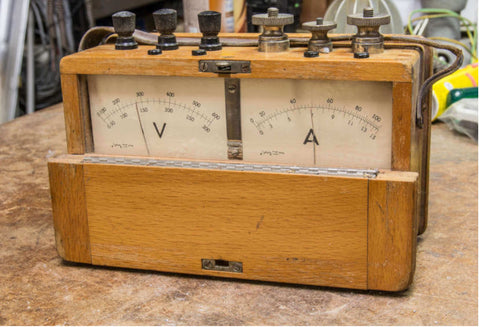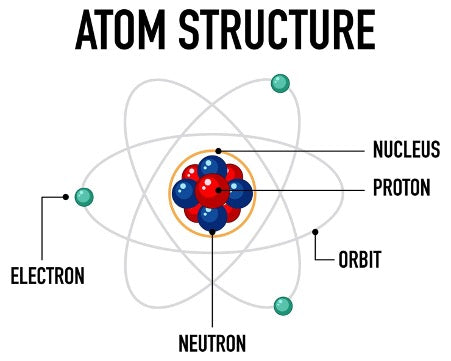Posted by Gomoto MediaWe get on our motorcycle, turn the key and the instrument panel lights up instantaneously. We thumb the ignition switch and the engine starts up. All these from a little box called a battery.When we reach home, we flick a few switches for the lights, TV, computer, fan/air-conditioner. We charge our phones so we can watch videos on TikTok.

Electricity is the cornerstone of our civilisation. Almost everything around us requires electricity to function. Ironically, many of us do not know what exactly is electricity, although it so essential that daily life is paralysed without it. So, here is a quick description - without becoming a physics dissertation, of course.

##### It is atomic• Electricity is a form of energy and begins at the atomic level. An atom is the smallest building block of all materials, including you and I.
• Each atom consists of neutrons, protons and electrons.
• Neutrons and protons occupy the centre of an atom, called the nucleus.
• Electrons orbit the nucleus, and they are responsible for electricity, hence the name.
• The protons account for the positive charge of the atom.
• Electrons are the negative charge of the atom.
• As an electron is lighter than a proton, thus there must be a certain number of electrons to balance the weight of the proton, thus forming an equilibrium.
• The state of equilibrium is called a neutral electrical condition i.e. net electrical charge of 0 (zero) or the atom's ground state.
• However, electrons can move freely and it is this movement that forms an electric current.
• We do this by adding or subtracting electrons.
• A surplus of electrons means the atom is negatively charged and becomes a negative ion.
• If there are fewer electrons to balance the protons, the atom becomes positively charged hence called a positive ion.
##### An electrical circuit• Nature seeks a balance for everything, including electricity. Thus, an electrical circuit allows for the movement of electrons when there is a charge imbalance.
• A circuit is complete when we connect a positively charged point to a negatively charged point.
• An easy way to visualise this is by filling a long tube with marbles then connecting each end with boxes.
• The whole contraption is then tilted up at the negative end.
• Each marble moving downwards from the negative end to the positive end is akin to an electron (although electrons are not influenced by gravity).
• Now let us consider the car's battery: The electrons move out from the battery's negative terminal (anode) through the cable (conductor) to the car's electrical components (resistance) and back into the battery via the positive terminal (cathode).
• A switch is a gateway for these electrons: Switching on means is completing the circuit, thereby allowing the electrons to move from the anode to cathode, and vice versa.
##### Amping it up• Named after André-Marie Ampère, the movement of electrons is called an electrical current.
• This rate of movement can be measured as the electrical unit Ampere or A, in short.
• Mathematically, 1A = 1C/1s, as in, 1 Coulomb in 1 second.
• Ampere measures the flow of electrons past a certain point in a circuit in one second. Coulomb, on the other hand, is the unit of electric charge, carried by 6.24 x 10^18 (6.24 quintillion or 6,240,000,000,000,000) electrons. Thus, a 2.1 A phone charger provides 2.1 times the numbers of electrons (C) per second.
• Thus, the answer to "what is electricity" is the flow of electrons.
##### High voltage• How about Voltage then? It is named after Alessandro Volta and measures electrical pressure.
• Imagine a water pump: The water pressure creates a flow of water. Similarly, electrical pressure creates a flow of electrons, hence an electric current.
• When we measure a battery's Voltage, we measure the strength of the current.
• When we measure a battery's Voltage at the anode and cathode, we are measuring the difference in pressure between these two terminals.
• In other words, Voltage is the measure of a power source's (be it a battery, generator, alternator, even lightning) potential work it can provide.
• This is why, a 1.5V battery cannot provide a strong enough flow of electrons to power a 12V car lightbulb.
• Mathematically, Voltage (V) = Coulomb (C) / Joule (J). For example, 14040 C / 9360 J = 1.5V.
##### Closing

Yes, it is a little difficult to wrap your mind over this at the beginning. It took us a while too! But trust us: You will understand it all after playing around with the bike's battery and electrical system.